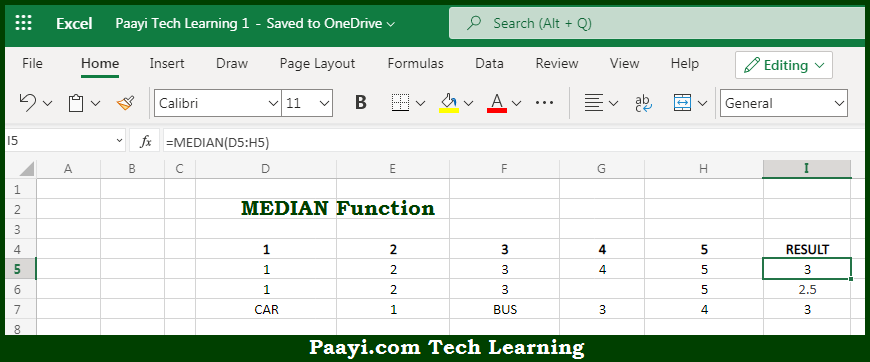# Learn How to Use Microsoft Excel MEDIAN Function

Written by | 0 Comments | 492 Views

In this article, you will learn how to use the Microsoft Excel MEDIAN function and its prime function in Microsoft Excel. You will also get to know the Microsoft Excel MEDIAN function return value and syntax with the help of some examples.

Microsoft Excel MEDIAN Function

The main purpose of the Microsoft Excel MEDIAN function is to get the median of a group of numbers. That implies, with the help of the MEDIAN function you can able to return the median that is the middle number, in a group of supplied numbers. Let's say for example, =MEDIAN(1,2,3,4,5,6,7) returns 4. So, with the help of the MEDIAN function, you can able to get the median from the group of numbers provided.

Return Value of MEDIAN Function

The return value will be the number representing the median.

Syntax of MEDIAN Function

=MEDIAN(number1, [number2], ...)

Where the arguments:

• number1: This is the number or cell reference that refers to numeric values.
• number2: This is the number or cell reference that refers to numeric values (optional).

## How to Use Microsoft Excel MEDIAN Function?So we know that Microsoft Excel MEDIAN function you can able to get the median of a group of numbers. That implies, with the help of the MEDIAN function you can able to return the median that is the middle number, in a group of supplied numbers. Let's say for example, =MEDIAN(1,2,3,4,5,6,7) returns 4. So, with the help of the MEDIAN function, you can able to get the median from the group of numbers provided.